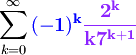# Example Comparing Two Infinite Series

Previous: Strategies for Convergence Testing

Next: Convergence Test Videos

In the following problem, we don't work out details on applying convergence tests. Rather, we discuss general strategies for convergence testing.

## Problem

State what convergence test you would use to determine whether the following series converge, and explain why:## Complete Solutions (and Discussion)

### Part a)

Because of the (-1)k term, the series is alternating, so we can try using the alternating series test (the ratio test could also be applied, but would require slightly more work to compute the ratio).

### Part b)

Unlike Part a), we do not have an alternating series, but the presence of constants raised to the power k suggests that the ratio test could be applied.

## Possible Challenges

### Applying the Divergence Test

You may have noticed that in parts a) and b) that the divergence test yields an inconclusive result. Because the divergence test is easy to apply, you may find that it is one of the first tests you tend to think about, but it isn't one that can tell us when a series converges.

### Not Recognizing The Form of the Series

Knowing how to classify a given infinite series requires being able to recognize what components of the series are helpful. If we consider Part a):The (-1)k term (highlighted in blue) suggests that we might try using the alternating series test. Other components (highlighted in purple) tell us what the outcome of the test will be.

Previous: Strategies for Convergence Testing

Next: Convergence Test Videos